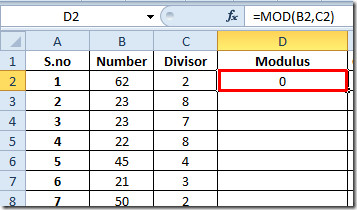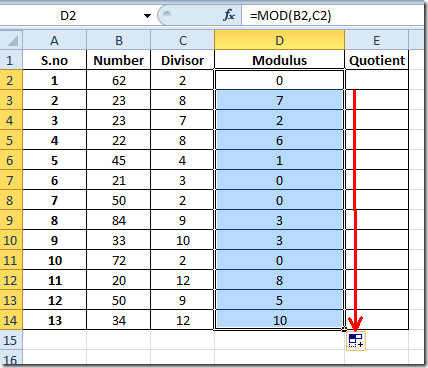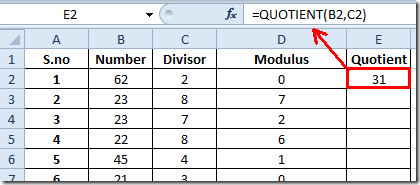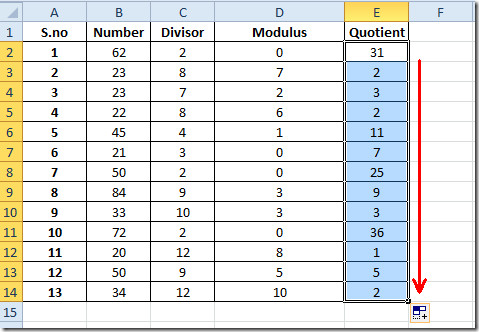# Find Modulus & Quotient In Excel 2010

Finding out modulus and quotient manually can become a tiresome process, especially when you are dealing with big numbers. Excel 2010 includes a build-in function to find out Modulus and Quotient instantly. These functions are dead-easy to use and take only two arguments for evaluation. The Modulus and Quotient functions require data range i.e., the cell location where values are residing or direct values to calculate modulus and the quotient, respectively. You can also copy or drag the cell containing the formula to calculate the modulus/quotient for the corresponding data set. It’s advisable to check the the divisor values before finding the modulus and quotient in order to get correct results for your data set. To get started, Launch Excel 2010 spreadsheet in which you need to find out modulus and quotient. For instance we have included a spreadsheet containing fields; S.no, Number and Divisor.

We need to find out modulus and quotient for values in Number field and Divisor field. For this we will add two more fields namely Modulus and Quotient adjacent to existing fields.

We will use the MOD function in the first row of Modulusfield. The syntax of MOD function is;

=MOD(number,divisor)

In function arguments, the number refers to the number that is to be divided and divisor refers to value, which will be considered as the divisor (a number which divides). We will be writing this function as;

=MOD(B2,C2)

In function arguments B2 and C2 refer to location of the cell where number and divisor value is residing. It will yield 0 as remainder (as 62 is completely divisible by 2).Drag the plus sign at the end of the cell towards the end of column to apply it over.For finding out quotient we will be writing a simple QUOTIENT function. The syntax of Quotient function is

=QUOTIENT(number,denominator)

In function arguments, the number refers to data value which will be divided and denominator refers to the value which divides the number. We will write this function as;

=QUOTIENT(B2,C2)

Where B2 and C2 are location of cells, where number and denominator value are residing. It will yield 31 (as 31 times 2 makes up 62).Apply the formula in the whole quotient field to find out quotient for all Number and Divisor field values.You can also check out our previously reviewed Excel functions; RANK.AVG and RANK.EQ, WORKDAY.INTL, LARGE, CEILING, TIME, ADDRESS ,FACT (factorial), MAX,MIN, MAXA, MINA and EXACT, Logical Functions, INFO, SUMSQ, DOLLAR, SUMPRODUCT, SUMIF, COUNTIF, VLOOKUP, HLOOKUP ,PMT, & LEN.

1.Sudhir Yadav says: